# Aleksandrov problem for isometric mappings

(diff) ← Older revision | Latest revision (diff) | Newer revision → (diff)

Let,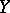be two metric spaces, with respective distances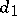,(cf. also Metric space). A mappingis defined to be an isometry if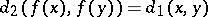for all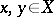. A mappingis said to preserve the distanceif for allwith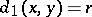one has. A.D. Aleksandrov has posed the problem whether the existence of a single preserved distance for some mappingimplies thatis an isometry (cf. [a1]).

Even if,are normed vector spaces, the above problem is not easy to answer (note that, in this case, taking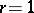is no loss of generality). For example, the following question has not been solved yet (as of 2000): Is a mappingfrom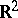to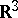preserving unit distance necessarily an isometry (cf. [a17])?

The discussion of Aleksandrov's problem under certain additional conditions on the given mapping preserving unit distance has led to several interesting and new problems (cf. [a14], [a15], [a16], [a18], [a17]).

The Aleksandrov problem has been solved for Euclidean spaces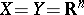: For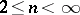, the answer is positive [a2], while for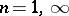, the answer is negative [a2], [a5], [a13]. In general normed vector spaces, the answer is positive for a mapping that is contractive, surjective and preserves the unit distance. The problem also has a positive solution whenandare strictly convex vector spaces, providedis a homeomorphism and the dimension ofis greater than(cf. [a12], [a20]).

The Aleksandrov problem has also been solved in some special cases of mappingswhich preserve two distances with an integer ratio greater than, withandstrictly convex vector spaces and the dimension ofgreater than(cf. [a3], [a16], [a17]). Furthermore, when,are Hilbert spaces and the dimension ofis greater than, a lot of work has been done (cf. [a21], [a22], [a23]; for example, whenpreserves the two distancesand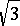; when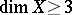andpreservesand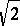, thenis an affine isometry, etc.).

Problems connected with stability of isometries as well as non-linear perturbations of isometries have been extensively studied in [a4], [a5], [a6], [a7], [a8], [a9], [a10], [a11].

How to Cite This Entry:
Aleksandrov problem for isometric mappings. Encyclopedia of Mathematics. URL: http://encyclopediaofmath.org/index.php?title=Aleksandrov_problem_for_isometric_mappings&oldid=17029
This article was adapted from an original article by Shuhuang Xiang (originator), which appeared in Encyclopedia of Mathematics - ISBN 1402006098. See original article